Geometry of Circles

INTRODUCTION

Circles are perhaps the most basic and simplest of figures.  They are defined as the locus of points equidistant from a center and enclose the greatest area for the smallest perimeter.

In early astronomy, models to predict the motion of the heavens were based on simple theorems involving circles.  This was a mathematical necessity for refining descriptions of simple harmonic motion rather than any religious or theological requirement notwithstanding after the fact musings on perfect geometric forms and the beauty God’s natural world.

THEOREMS

Some properties of triangles and polygons inscribed in a circle include the following.

For fixed points of “A” and “B” on the circumference of the circle, all angles from another point on the circumference are equal.  That is to say, we have for all such angles θ = φ . This should be somewhat surprising that as the point, i.e. c1 or c2, forming the angle moves around the circumference of the circle, the angle remains unchanged.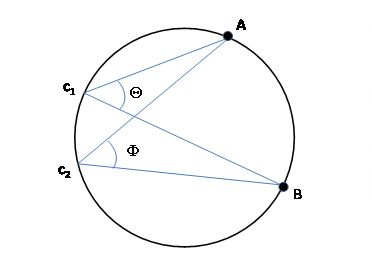For fixed points of “A” and “B” on the circumference of the circle, the angle from any point “C” on the circumference φ is equal to twice the angle θ from the center of the circle or θ = 2 φ .  Note that the angle θ always faces away from φ and thus varies from 0 to 360° or 2π radians while the total variation in φ is exactly half of that.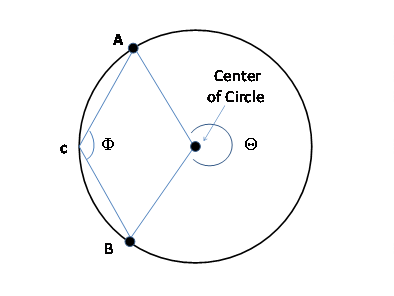For fixed points of “A” and “B” on the circumference of the circle, the line between them is a diameter of the circle if that line goes through the center.  Then for any inscribed triangle including the arbitrary point “C” the angle φ is a right angle or φ = 90° or π/2 radians.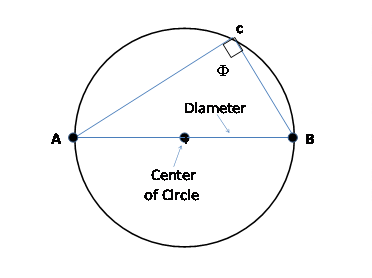For an inscribed quadrilateral with points “A”, “B”, “C”, and “D” on the circumference of a circle, the opposite angles are supplementary.  That is

θ + φ = Ψ + Ω =180° or π radians.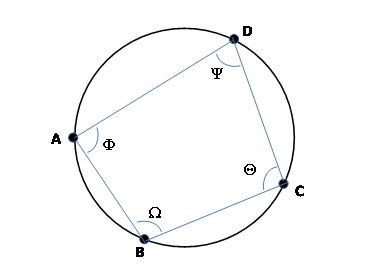For an inscribed quadrilateral with points “A”, “B”, “C”, and “D” on the circumference of a circle, the lengths of chords are as follows. This was known to Ptolemy (c. 100-170 A.D.) in the first century A.D.

a*b + c*d = e*f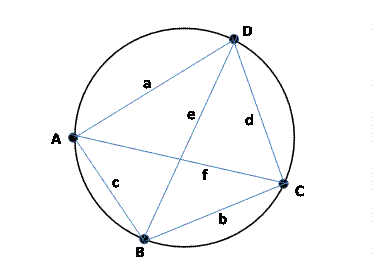And when the inscribed quadrilateral is a rectangle, this reduces to the Pythagorean Theorem as

a*a + c*c = e*e = f*f.

The tangent line to a circle makes a right angle with the radius to the tangent point “A” on the circumference.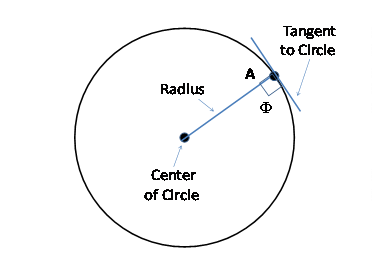Note that the angles at “A” and “B” are the same as at “C” with respect to the tangent to the circle at that point.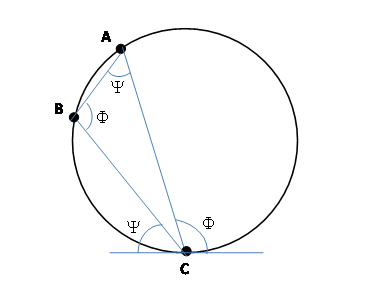If the circle is of radius “r”, then from the right angles shown, we have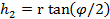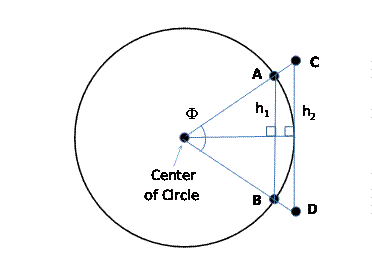The length of the interior inscribed chord from “A” to “B” is simply twice “h1” and the exterior from “C” to “D” is twice “h2”.

To construct either an inscribed or circumscribed regular polygon of “n” sides, the angle φ must be an integral multiple of 360° or 2π radians or φ=360°/n.   For instance if n=6, we have an inscribed and circumscribed hexagon with φ=60° or π/3 radians.We can write expressions for the perimeters of an inscribed and circumscribed regular polygon of “n” sides.  Please note that these bound the circumference of a circle (of radius equal to one) as follows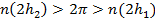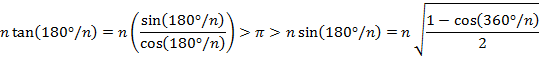A table of the perimeter of circumscribed and inscribed polygons of “n” sides as compared to π is given below

 n sides Circumscribed Perimeter/2 π Inscribed Perimeter/2 10 3.2492 3.1416 3.0902 20 3.1677 3.1416 3.1287 30 3.1531 3.1416 3.1359 40 3.1481 3.1416 3.1384 50 3.1457 3.1416 3.1395 60 3.1445 3.1416 3.1402 70 3.1437 3.1416 3.1405 80 3.1432 3.1416 3.1408 90 3.1429 3.1416 3.1410 100 3.1426 3.1416 3.1411 110 3.1424 3.1416 3.1412 120 3.1423 3.1416 3.1412

HISTORICAL TABLES

The Alfonsine Tables were the first set of trigonometric and astronomical tables used to predict eclipses and the positions of the planets prepared in Christian Europe.  The table entries began in the year 1252 A.D. which was the first year of the reign of King Aphonso X (1221-1284 A.D) of Leon and Castile to whom they were dedicated .  They were centered on the meridian of Toledo, Spain and needed slight adjustments for other locales.

Despite often whimsical speculation, they were in fact derived entirely from the original and unmodified model of the Greco-Roman mathematician Ptolemy (c. 100-170 A.D.) which remained more accurate than all intervening and ineffectual attempts to improve it.  Or perhaps this also reflects the difficulty in disseminating little known and little improved thoughts written in Arabic.   In any event, the original work was in Castilian Spanish but about 1320 A.D. a Latin version was prepared in Paris, France after which it gained a wider audience.   The first printed version only appeared in 1483.

Other tables were prepared by John Muller of Konigsberg (1436-1476 A.D.) who wrote under the pseudonym of “Regiomontantus”.   He popularized the mathematics of solid spherical geometry to predict heavenly motion using the original Ptolemaic model with his publication of a new translation of the “Almagest”.  One notable feature was an extensive table of the inscribed “chords” of a circle which are effectively the trigonometric “sine” function.

Copernicus (1473-1543 A.D.) purchased a copy of the Alfonsine Tables while at the University of Krakow which still survives with his annotations.  Pages were sold separately by the printer as individual loose leaves and it was necessary for the buyer to have them separately bound into a single volume.

The first astronomical calculations using a model different from Ptolemy were the Prutenic tables dedicated to Albert I, Duke of Prussia.  These were prepared by Erasmus Reinhold and published in 1551.  They were based on the slightly less accurate heliocentric theory of Copernicus.  Ironically, while this new model was more complex having more epicycles than Ptolemy, the calculations removed several mathematical artifices and were thus easier to use especially as they eliminated Ptolemy’s “equant.”  In any event, they also included redundant data to update the older Alfonsine tables based on the original unmodified Ptolemaic model.

The Copernican model also suffered from an inaccurate estimate of the average topical year.   Interestingly, the length of one year to the next varies typically by about 30 minutes due to the small gravitational influences of the moon, sun, and planets.  The long term average is 365 days 5 hours 49 minutes and 45 seconds which was a number finally and first calculated by Kepler.

Significant accuracy, of more than an order of magnitude, was only achieved with the Rudolphine tables prepared by Johannes Kepler in 1625 and finally published in 1627 in Prague.  These were based on Tycho Brahe’s lifetime of observations incredibly accurate to one arc minute or 1/60th of a degree.  To date, these remain by far the most accurate naked-eye astronomical records made without a telescope.   And it was only this accuracy that enabled Johannes Kepler to develop the modern heliocentric model.

REFERENCES

1.  A quote by King Alphonso X, the Wise, was that “If God had consulted me before embarking on the Creation, I would have suggested something simpler.”